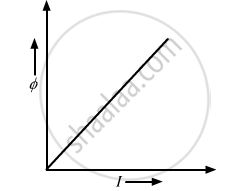# The Current Flowing Through an Inductor of Self Inductance L is Continuously Increasing. Plot a Graph Showing the Variation of Magnetic Flux Versus the Current - Physics

The current flowing through an inductor of self inductance L is continuously increasing. Plot a graph showing the variation of

Magnetic flux versus the current

#### Solution

Since ϕ = L I

where,   I = Strength of current through the coil at any time
ϕ =  Amount of magnetic flux linked with all turns of the coil at that time
and, L = Constant of proportionality called coefficient of self inductionConcept: Magnetic Flux
Is there an error in this question or solution?
2013-2014 (March) Delhi Set 1

Share